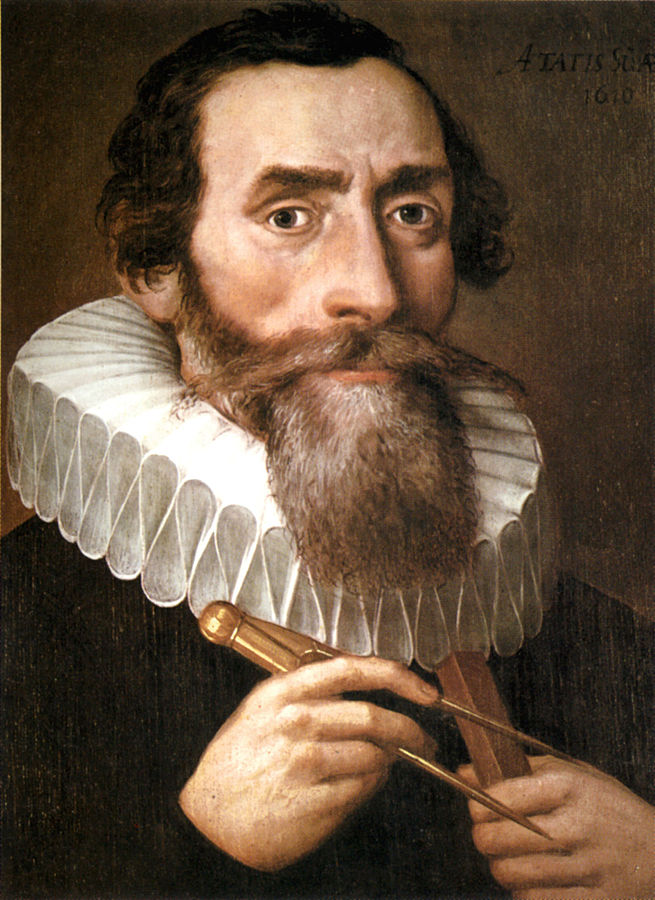Gravity and Orbits

Basic Orbits

Why circles?Copernicus - woodcut attributed to Christoph Murer, from Nicolas Reusner's Icones (1587)

Copernicus (1473-1543)The Copernican System consisted of circular orbits centered around the sun.

Johannus KeplerGerman mathematician, astronomer, and astrologer. [1571-1630] Kepler was Tycho's assistant and was believed in the Copernican system.

Kepler (1571-1630)

Kepler's 3 laws of orbiting bodies

1. A planet orbits the sun in an ellipse. The Sun is at one focus of that ellipse.
2. A line connecting a planet to the Sun sweeps out equal areas in equal times
3. The square of a planet's orbital period is proportional to the cube of the average distance between the planet and the sun: $P^2 \propto a^3$.

1st Law: Ellipses

Mars' orbit [$e = 0.0934$] looks a lot like a circle.

2nd Law: Equal Areas in Equal TimesThe blue areas in these figures will be the same if $t_2 - t_1$ is the same.

2nd Law: ProofAn orbiting object moves through angle $\Delta \theta$ in a given time: $\Delta t$

Characteristics of Different Orbits

Circular:

The eccentricity $\epsilon = 0$, and the radius $r$ is constant.

Both foci are located at the center.

These don't really exist in nature but are just mathematical possibilities

Elliptical

Are bound and closed orbits where the eccentricity is $0 \lt \epsilon \lt 1$

Have the gravitational center at the principle focus

$r_p = a(1 - \epsilon)$ and $r_a = a(1+ \epsilon)$

Shape is determined by any of the following pairs:

 $E$ $l$ $\epsilon$ $r_p$ $a$ $b$

Parabolic

$\epsilon = 1$

Like circules, these don't actually occur but are just mathematical possibilities.

Hyperbolic

$\epsilon > 1$

Bertrand's Theorem

The only central-force potentials $U(r)$ for which all bounded orbits are closed are:

1. Gravity: $U \propto \frac{1}{r}$
2. Springs: $U \propto r^2$

Hohmann Transfer Orbit

The semi-major axis of the transfer orbit: \begin{equation} a_\textrm{transfer} = \frac{r_e+r_m}{2} \end{equation}